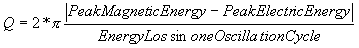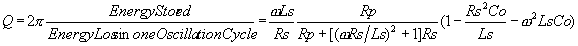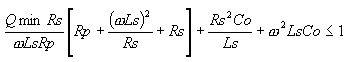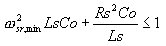HOMEHIGH Q CIRCULAR SPIRAL INDUCTORS OPTIMIZATION

 APPLICATION NOTE USM 106 Author: Mihaela Radulescu, M Physic. Sc. member technical staff, SEMICONIX CORPORATION Quality factor (Q) The efficiency of an inductor is measured by its Q, which is limited by the parasitics. The energy storage and loss mechanisms in an inductor can be described by an equivalent energy model (Fig.1), where Ls, Rs, Rp, and Co represent the overall inductance, conductor loss, substrate loss, and overall capacitance respectively.Fig.1 Equivalent energy model representing the energy storage and loss mechanisms in a monolithic inductor. Note that Co = Cp + Cs. For an inductor, only the energy stored in the magnetic field is of interest. Any energy stored in the inductor's electric field, because of some inevitable parasitic capacitances in a real inductor, is a loss. Hence, Q is proportional to the net magnetic energy stored, which is equal to the difference between the peak magnetic and electric energies. An inductor is at self-resonance when the peak magnetic and electric energies are equal. Therefore, Q vanishes to zero at the self-resonance frequency. Above the self-resonance frequency, no net magnetic energy is available from an inductor to any external circuit. To be more detail, the inductor Q can be defined as,(1) Combining the energy terms in Fig. 2 according to the fundamental definition of Q yieldswhere wLs/Rs accounts for the magnetic energy stored and the ohmic loss in the spiral conductor. The second term is the substrate loss factor representing the energy dissipated in the substrate. The last term is the self-resonance factor describing the reduction in Q due to the increase in the peak electric energy with frequency and the vanishing of Q at the self-resonant frequency. An important effect that degrades the Q is the current crowding effect. For a typical spiral, the current crowding is a strong function of frequency and the resistance increases at higher than linear rate. This causes the Q to go down in a concave. The specification for minimum quality factor (Q³Qmin) can be written as a posynomial inequality in the design variables and Qmin:(3) We can therefore specify a minimum required quality factor. We may also maximize the quality factor by maximizing Qmin subject to constraint (3). Minimum self-resonance frequency. The self-resonance frequency wsr is the frequency at which the quality factor Q is zero A condition on minimum self-resonance frequency wsr ³wsr;min, can be written as the posynomial inequality(4) Therefore we can handle a specification on minimum self-resonance frequency and we can maximize the self-resonance frequency (by maximizing subject to constraint (4) ). Optimal design of inductors A common problem in inductor design is to maximize the quality factor for a given inductance value and for a minimum self-resonance frequency. Other constraints may be added (such as the minimum spacing and turn width, the maximum area available, the maximum parallel capacitance, etc.). The point is that the design problem can be formulated as a geometric program. Geometry constraints The monomial inequalities W>Wmin and s>smin handle the processing constraints that limit the minimum feature size. The inductor area can be constrained or minimized using the monomial inequality, p(Dout/2)2<=Amax. Metal width and spacing The spiral traces should be as wide as possible until the skin effect becomes significant. The increase of the metal width results in a rise of Q because the resistance of the inductor decreases and the inductance remains the same. The spacing between the metal lines should be as small as possible. Increasing the spacing decreases the total inductance because of the decreasing mutual inductance. It also increases the series resistance and the total area. If the metal spacing is increased, the Q will increase slightly and the inductance will decrease. Spiral radius The spiral radius is a very complex parameter to select. As the radius increases, so does the Q. This assumption is valid for small radius. For values greater than 90 to 100µm the losses induced by the eddy currents are heavy, which degrades the quality factor. Another point should be taken into account when selecting the spiral radius. As the radius increases, the metal area shared between the spiral and the substrate grows and increases the parasitic capacitance between the substrate and the spiral. This reduces the resonant frequency of the spiral. Therefore, the radius should be elected so that the frequency of operation of the spiral is not close to the resonance. Inductors with narrower lines have higher inductance and lower shunt capacitance per line compared to inductor with wider lines. Unlike the narrow-width spirals, wide-strip narrow-spacing multi-turn spirals suffer from eddy current and proximity effects especially in the loops closest to the center of spiral; however, the wide strips and the associated metal thickness together help reduce the DC resistance significantly compared to narrow-width spiral inductors. Given the above arguments, if a wide-strip and narrow-strip inductor were designed in the same two-dimensional area with the same line-to-line spacings, the narrow-strip spiral would have a much higher inductance, similar capacitance, lower SRF, and similar resistance (AC+DC) as compared to the wide-strip inductor.
 US MICROWAVES www.usmicrowaves.com Tel:(408)986-8026 Fax:(408)986-8027